Скачать презентацию Presentation Outline Introduction and general clustering techniques Motivation

50cae22a69cf2892cca3b71bf44b9d0d.ppt

• Количество слайдов: 53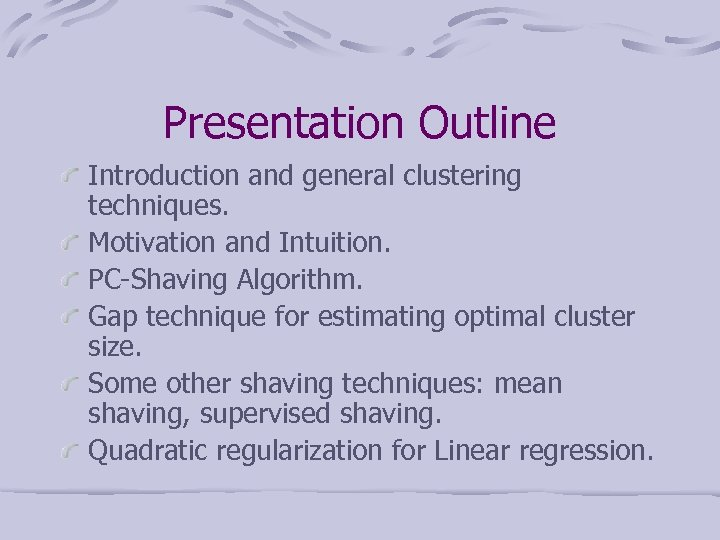Presentation Outline Introduction and general clustering techniques. Motivation and Intuition. PC-Shaving Algorithm. Gap technique for estimating optimal cluster size. Some other shaving techniques: mean shaving, supervised shaving. Quadratic regularization for Linear regression.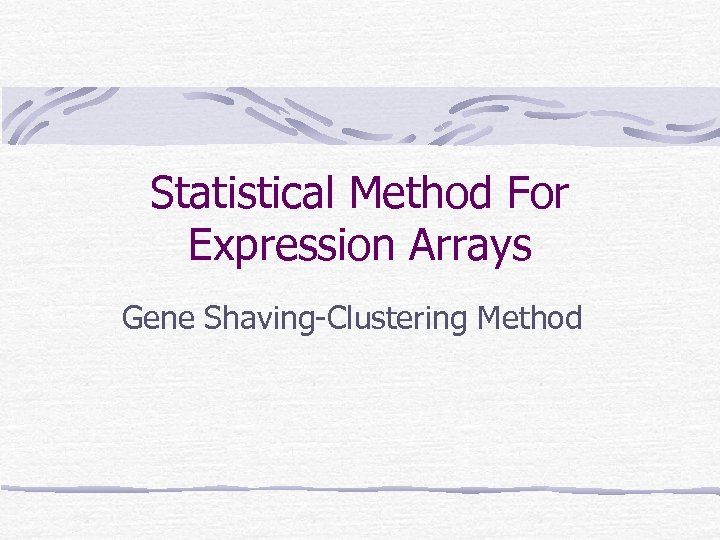Statistical Method For Expression Arrays Gene Shaving-Clustering Method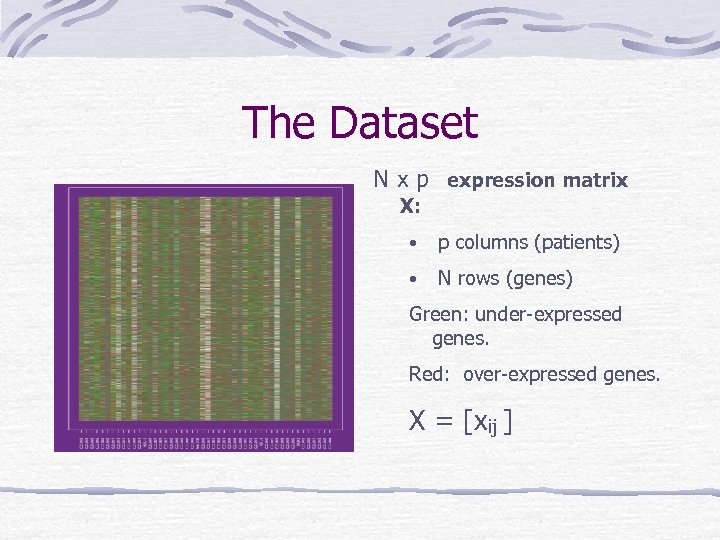The Dataset N x p expression matrix X: • p columns (patients) • N rows (genes) Green: under-expressed genes. Red: over-expressed genes. X = [xij ]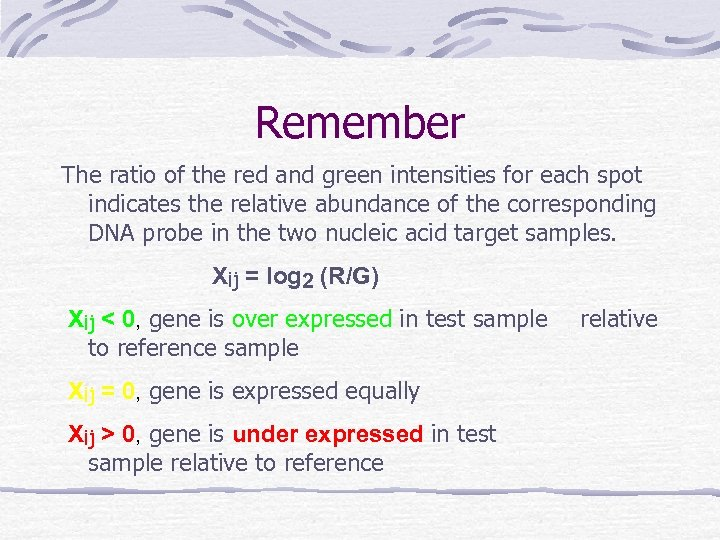Remember The ratio of the red and green intensities for each spot indicates the relative abundance of the corresponding DNA probe in the two nucleic acid target samples. Xij = log 2 (R/G) Xij < 0, gene is over expressed in test sample to reference sample Xij = 0, gene is expressed equally Xij > 0, gene is under expressed in test sample relative to reference relative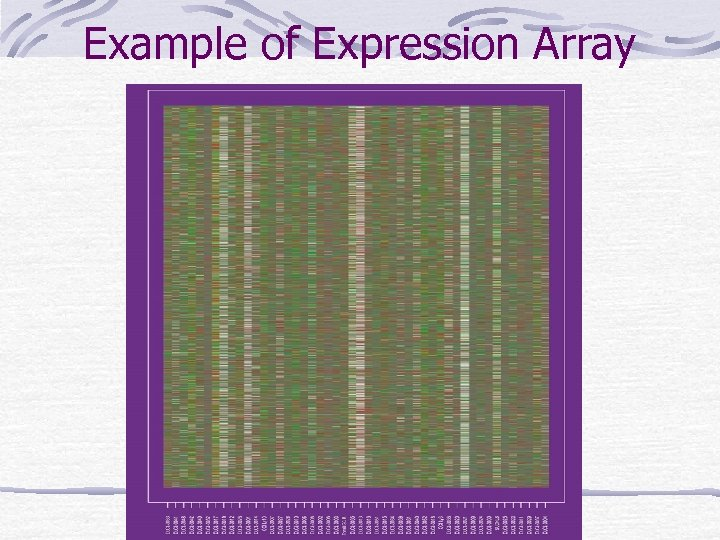Example of Expression Array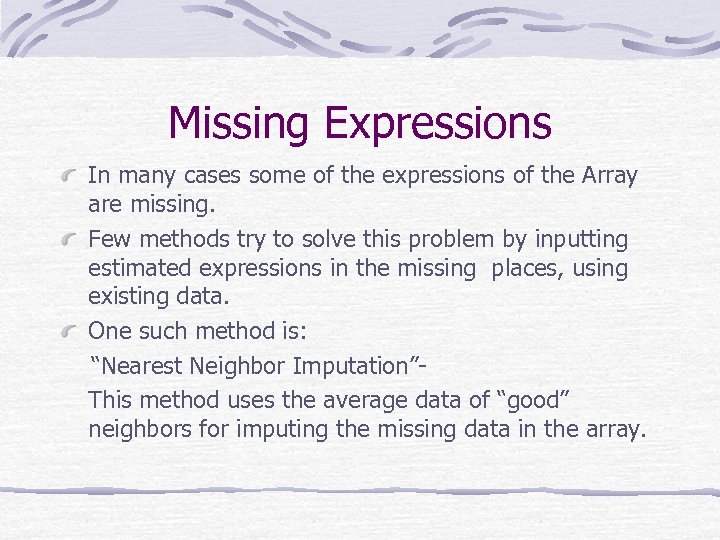Missing Expressions In many cases some of the expressions of the Array are missing. Few methods try to solve this problem by inputting estimated expressions in the missing places, using existing data. One such method is: “Nearest Neighbor Imputation”This method uses the average data of “good” neighbors for imputing the missing data in the array.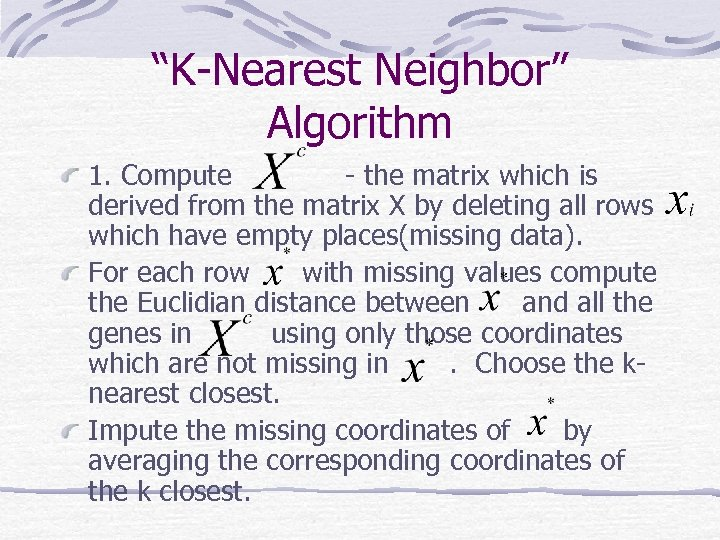“K-Nearest Neighbor” Algorithm 1. Compute - the matrix which is derived from the matrix X by deleting all rows which have empty places(missing data). For each row with missing values compute the Euclidian distance between and all the genes in using only those coordinates which are not missing in. Choose the knearest closest. Impute the missing coordinates of by averaging the corresponding coordinates of the k closest.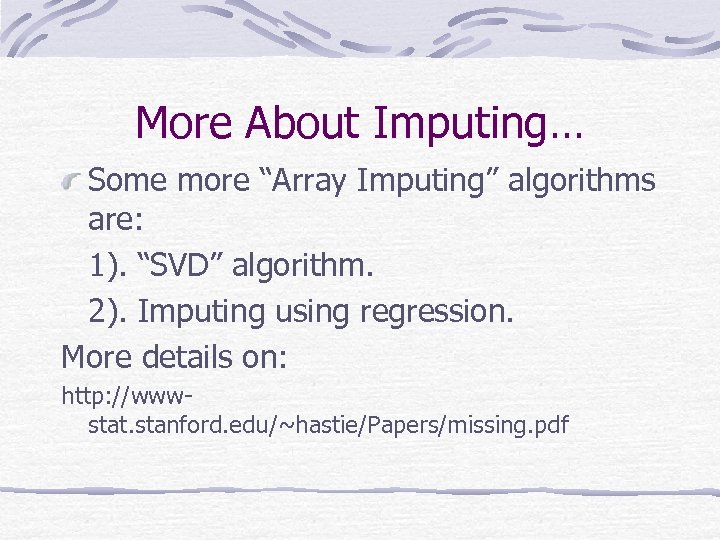More About Imputing… Some more “Array Imputing” algorithms are: 1). “SVD” algorithm. 2). Imputing using regression. More details on: http: //wwwstat. stanford. edu/~hastie/Papers/missing. pdf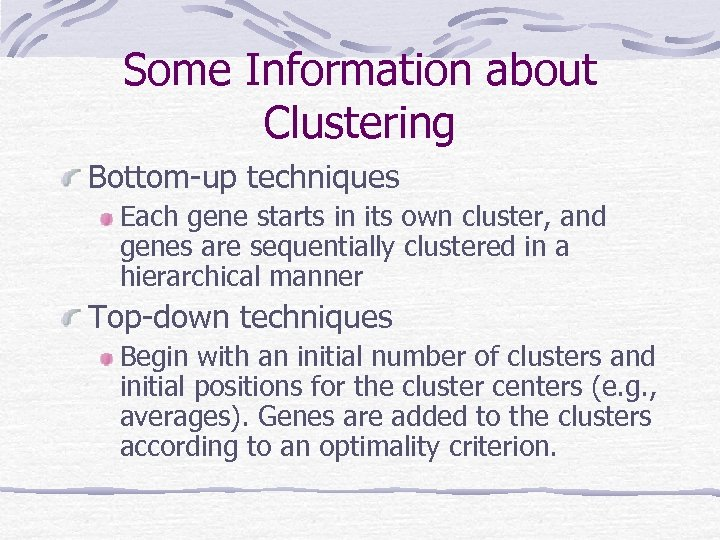Some Information about Clustering Bottom-up techniques Each gene starts in its own cluster, and genes are sequentially clustered in a hierarchical manner Top-down techniques Begin with an initial number of clusters and initial positions for the cluster centers (e. g. , averages). Genes are added to the clusters according to an optimality criterion.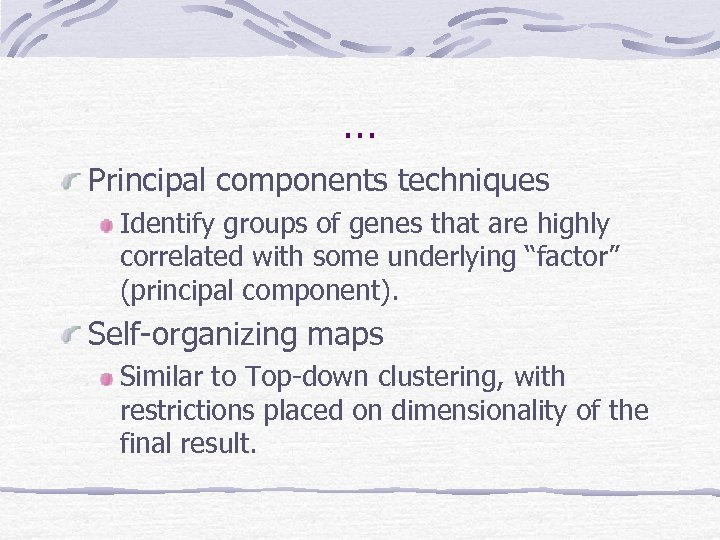… Principal components techniques Identify groups of genes that are highly correlated with some underlying “factor” (principal component). Self-organizing maps Similar to Top-down clustering, with restrictions placed on dimensionality of the final result.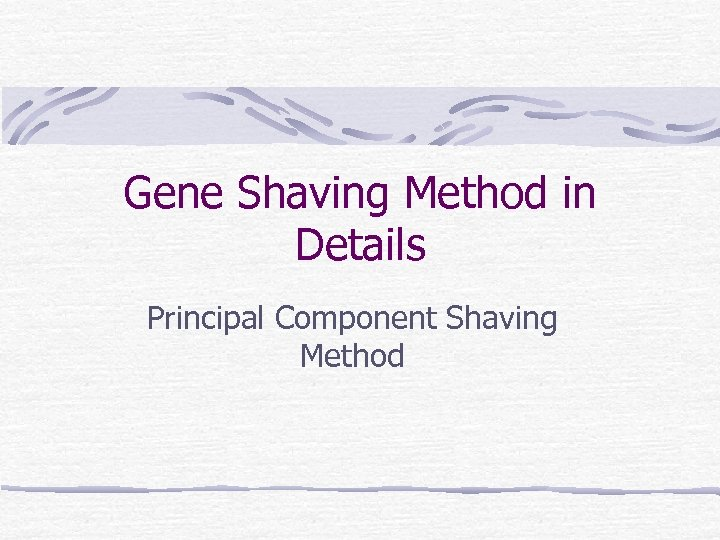Gene Shaving Method in Details Principal Component Shaving Method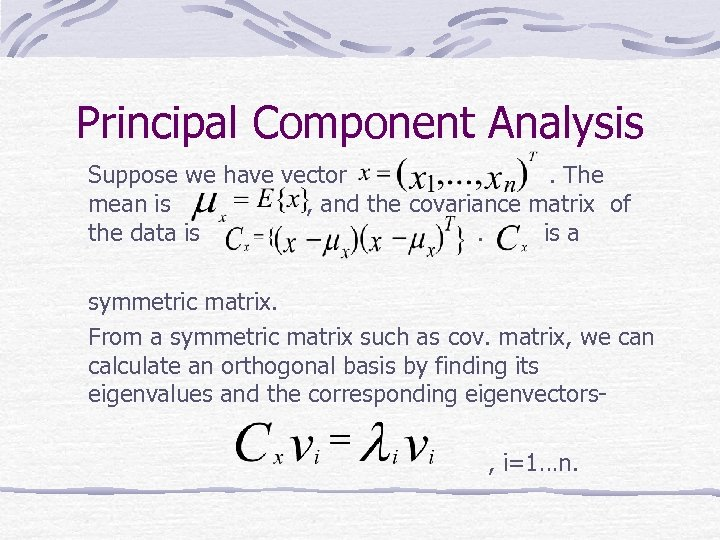Principal Component Analysis Suppose we have vector. The mean is , and the covariance matrix of the data is. is a symmetric matrix. From a symmetric matrix such as cov. matrix, we can calculate an orthogonal basis by finding its eigenvalues and the corresponding eigenvectors, i=1…n.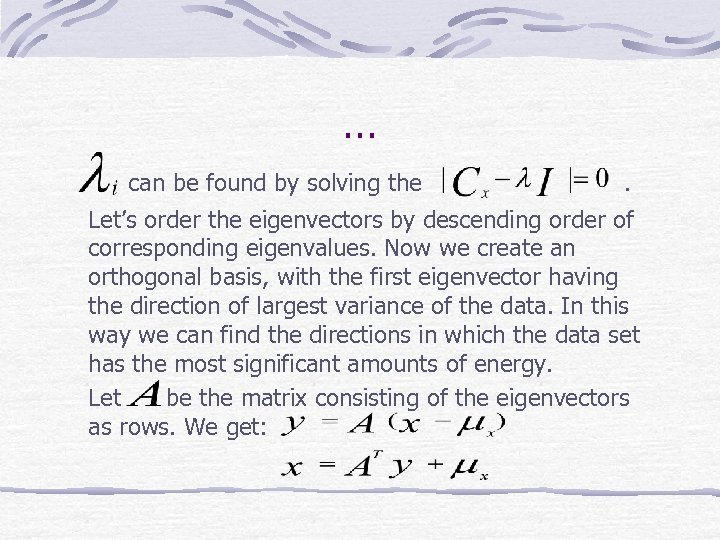… can be found by solving the . Let’s order the eigenvectors by descending order of corresponding eigenvalues. Now we create an orthogonal basis, with the first eigenvector having the direction of largest variance of the data. In this way we can find the directions in which the data set has the most significant amounts of energy. Let be the matrix consisting of the eigenvectors as rows. We get: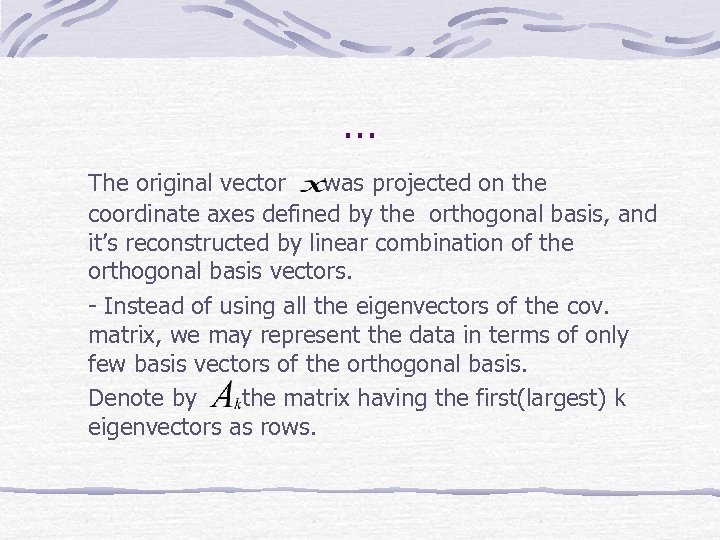… The original vector was projected on the coordinate axes defined by the orthogonal basis, and it’s reconstructed by linear combination of the orthogonal basis vectors. - Instead of using all the eigenvectors of the cov. matrix, we may represent the data in terms of only few basis vectors of the orthogonal basis. Denote by the matrix having the first(largest) k eigenvectors as rows.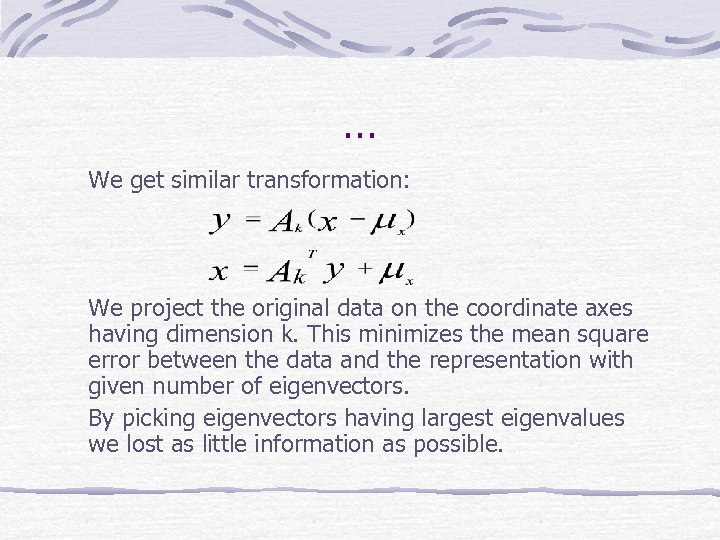… We get similar transformation: We project the original data on the coordinate axes having dimension k. This minimizes the mean square error between the data and the representation with given number of eigenvectors. By picking eigenvectors having largest eigenvalues we lost as little information as possible.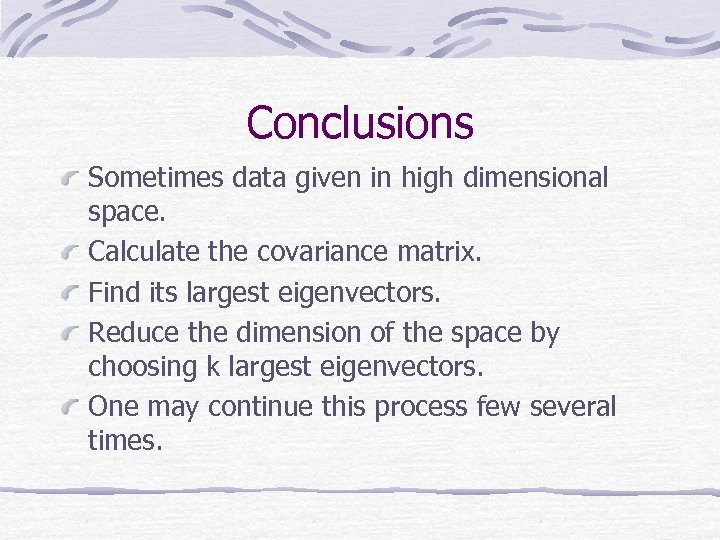Conclusions Sometimes data given in high dimensional space. Calculate the covariance matrix. Find its largest eigenvectors. Reduce the dimension of the space by choosing k largest eigenvectors. One may continue this process few several times.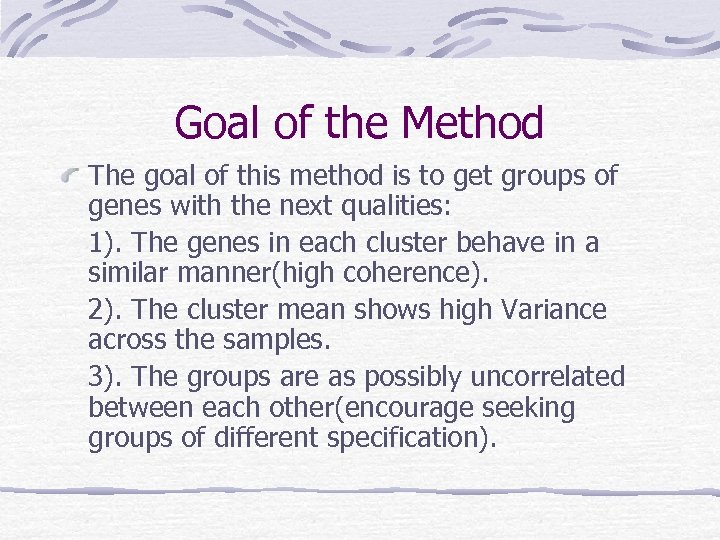Goal of the Method The goal of this method is to get groups of genes with the next qualities: 1). The genes in each cluster behave in a similar manner(high coherence). 2). The cluster mean shows high Variance across the samples. 3). The groups are as possibly uncorrelated between each other(encourage seeking groups of different specification).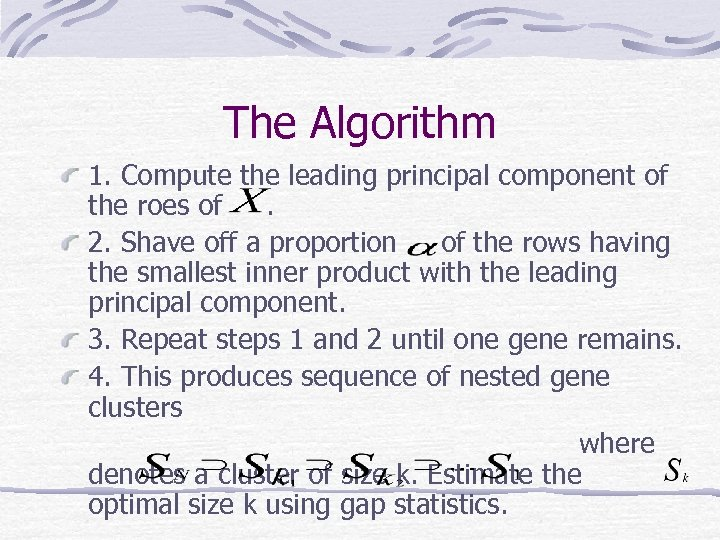The Algorithm 1. Compute the leading principal component of the roes of. 2. Shave off a proportion of the rows having the smallest inner product with the leading principal component. 3. Repeat steps 1 and 2 until one gene remains. 4. This produces sequence of nested gene clusters where denotes a cluster of size k. Estimate the optimal size k using gap statistics.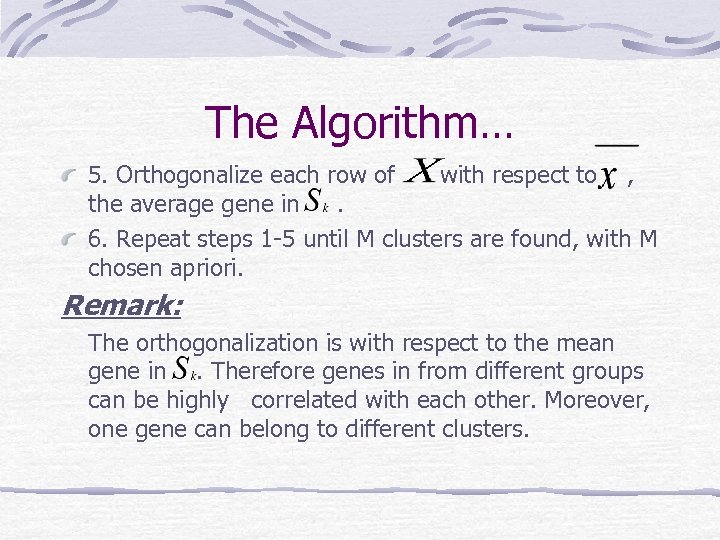The Algorithm… 5. Orthogonalize each row of with respect to , the average gene in. 6. Repeat steps 1 -5 until M clusters are found, with M chosen apriori. Remark: The orthogonalization is with respect to the mean gene in. Therefore genes in from different groups can be highly correlated with each other. Moreover, one gene can belong to different clusters.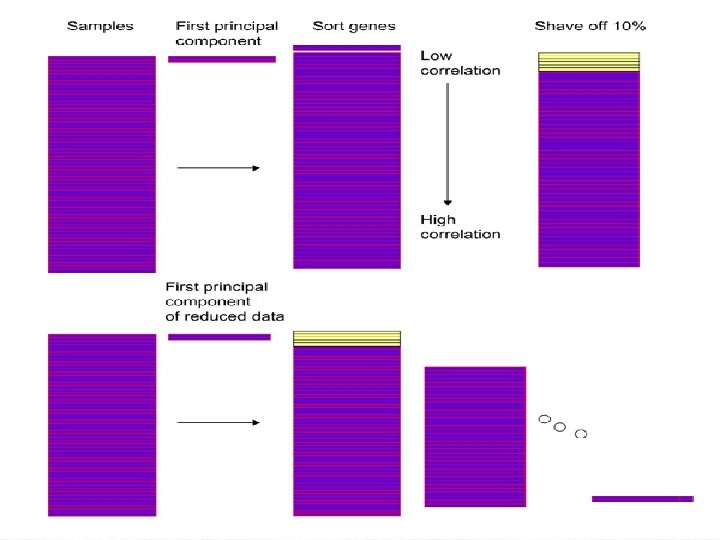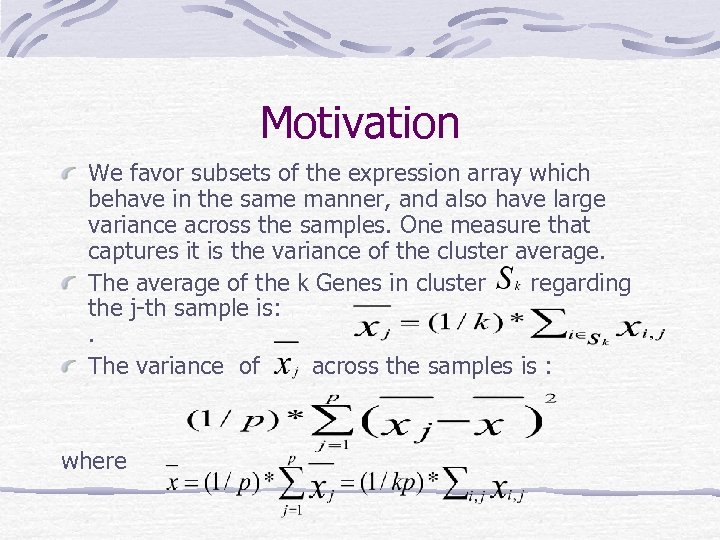Motivation We favor subsets of the expression array which behave in the same manner, and also have large variance across the samples. One measure that captures it is the variance of the cluster average. The average of the k Genes in cluster regarding the j-th sample is: . The variance of across the samples is : where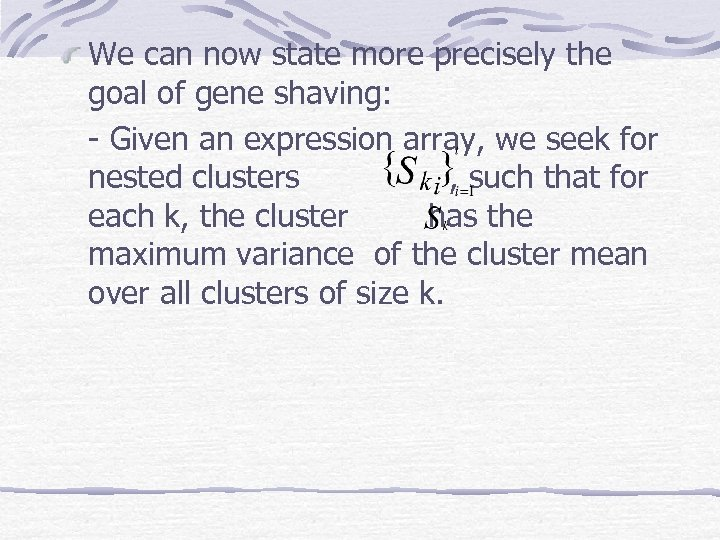We can now state more precisely the goal of gene shaving: - Given an expression array, we seek for nested clusters , such that for each k, the cluster has the maximum variance of the cluster mean over all clusters of size k.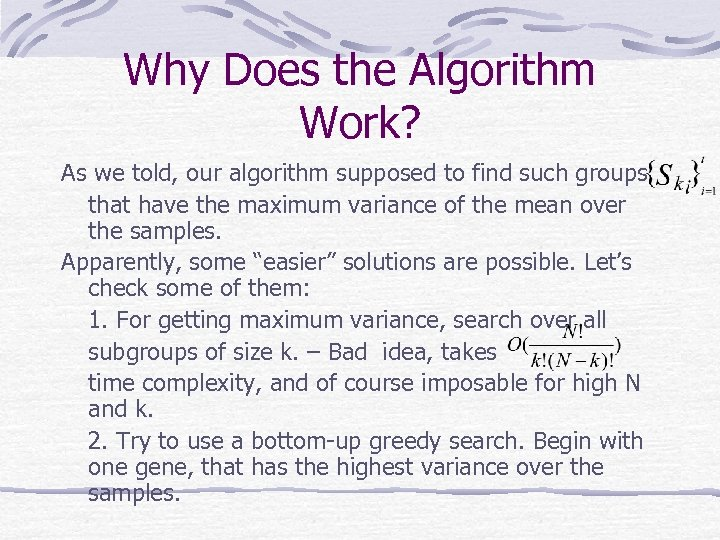Why Does the Algorithm Work? As we told, our algorithm supposed to find such groups that have the maximum variance of the mean over the samples. Apparently, some “easier” solutions are possible. Let’s check some of them: 1. For getting maximum variance, search over all subgroups of size k. – Bad idea, takes time complexity, and of course imposable for high N and k. 2. Try to use a bottom-up greedy search. Begin with one gene, that has the highest variance over the samples.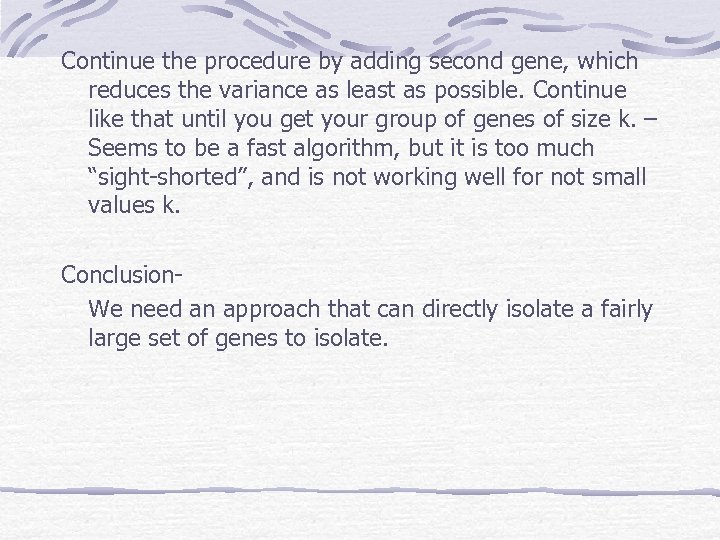Continue the procedure by adding second gene, which reduces the variance as least as possible. Continue like that until you get your group of genes of size k. – Seems to be a fast algorithm, but it is too much “sight-shorted”, and is not working well for not small values k. Conclusion. We need an approach that can directly isolate a fairly large set of genes to isolate.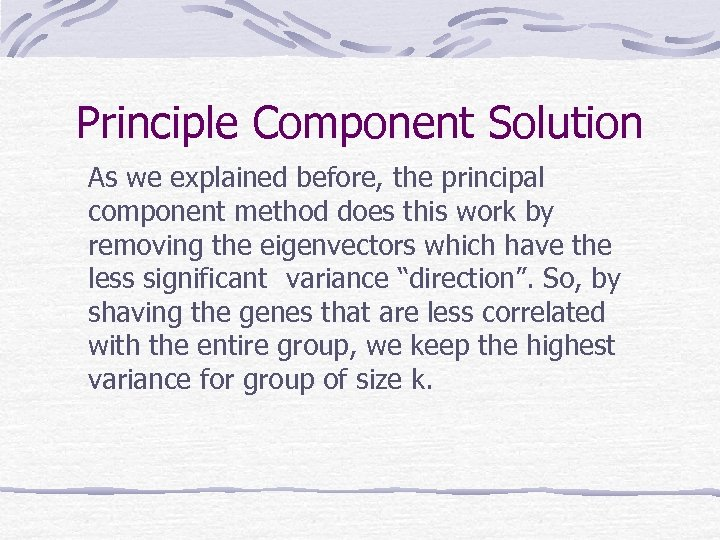Principle Component Solution As we explained before, the principal component method does this work by removing the eigenvectors which have the less significant variance “direction”. So, by shaving the genes that are less correlated with the entire group, we keep the highest variance for group of size k.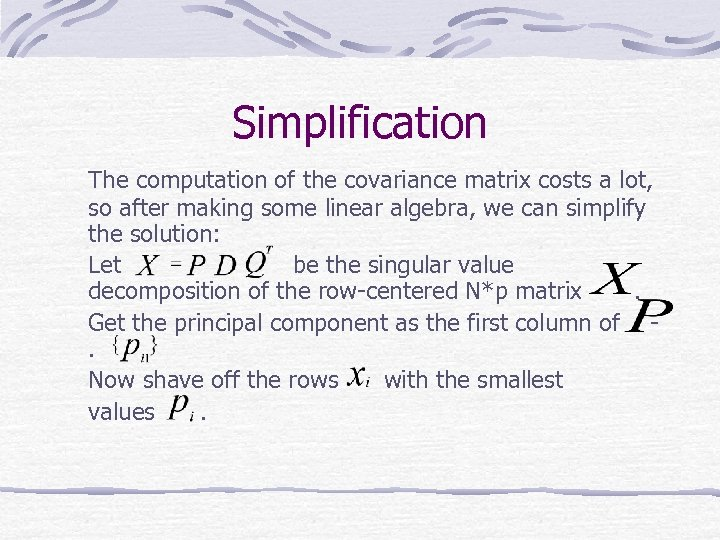Simplification The computation of the covariance matrix costs a lot, so after making some linear algebra, we can simplify the solution: Let be the singular value decomposition of the row-centered N*p matrix. Get the principal component as the first column of. Now shave off the rows with the smallest values.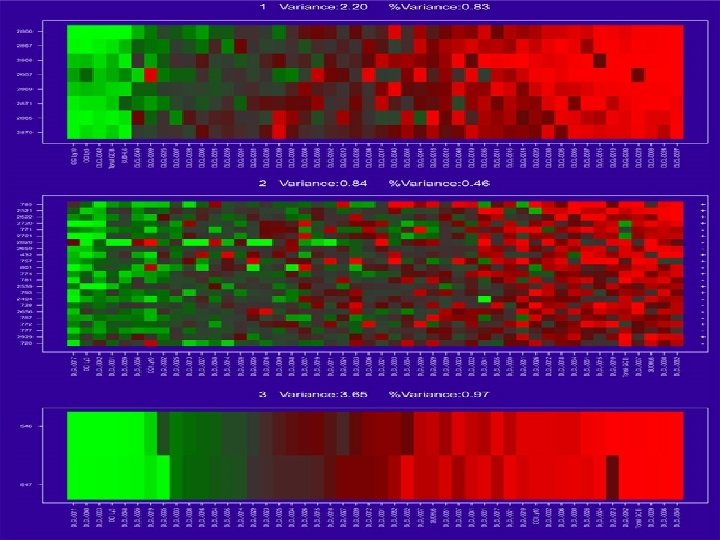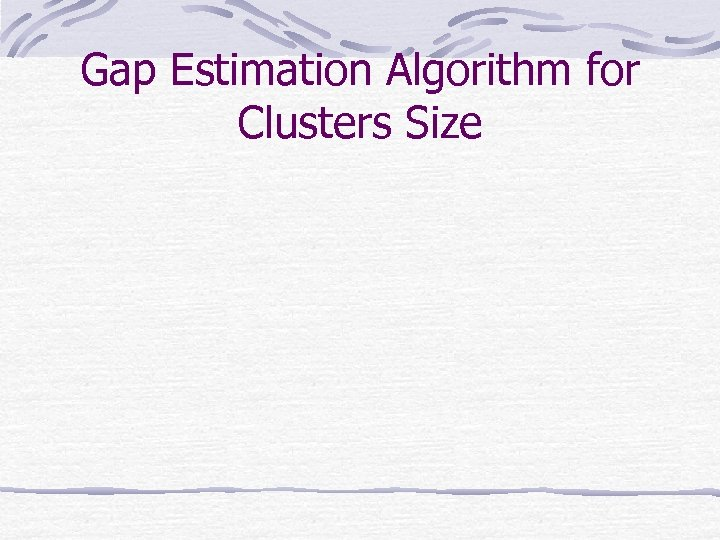Gap Estimation Algorithm for Clusters Size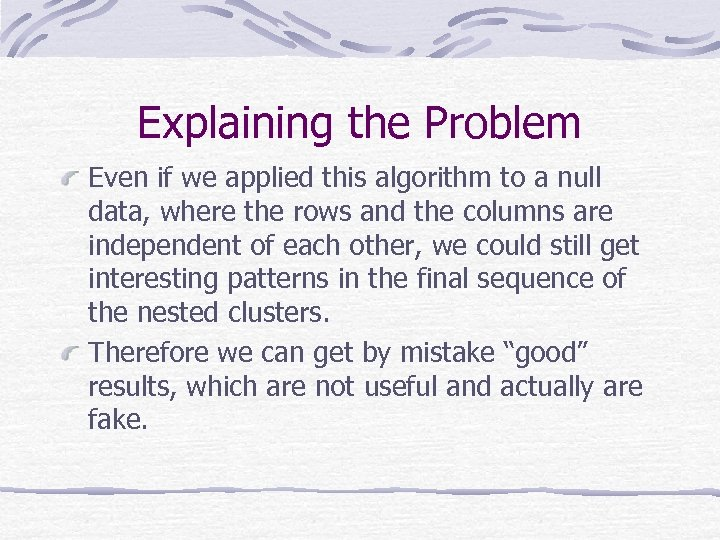Explaining the Problem Even if we applied this algorithm to a null data, where the rows and the columns are independent of each other, we could still get interesting patterns in the final sequence of the nested clusters. Therefore we can get by mistake “good” results, which are not useful and actually are fake.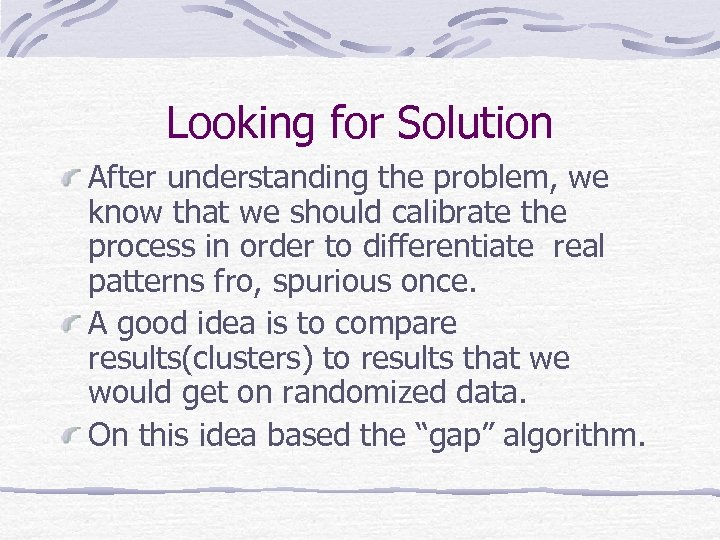Looking for Solution After understanding the problem, we know that we should calibrate the process in order to differentiate real patterns fro, spurious once. A good idea is to compare results(clusters) to results that we would get on randomized data. On this idea based the “gap” algorithm.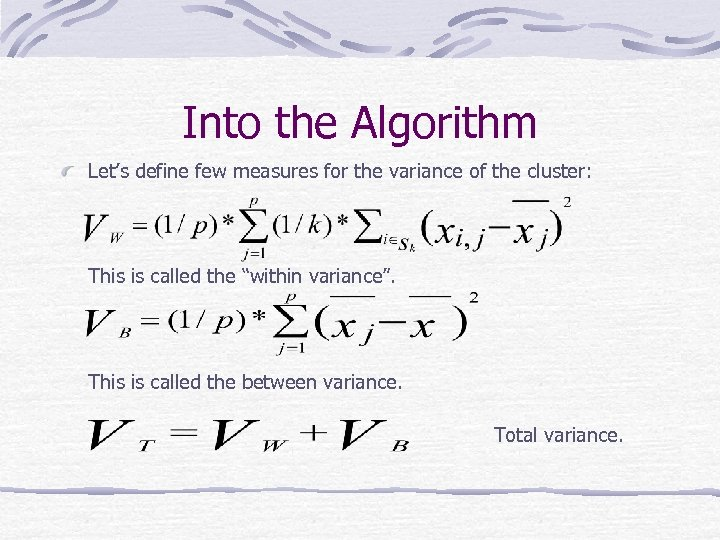Into the Algorithm Let’s define few measures for the variance of the cluster: This is called the “within variance”. This is called the between variance. Total variance.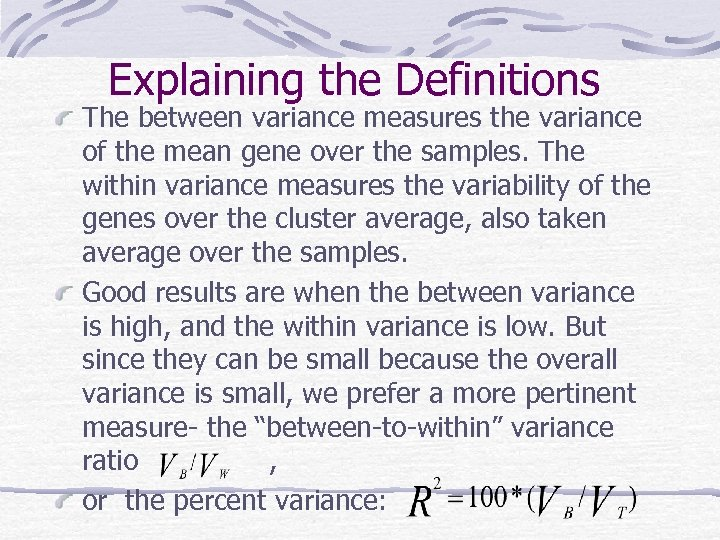Explaining the Definitions The between variance measures the variance of the mean gene over the samples. The within variance measures the variability of the genes over the cluster average, also taken average over the samples. Good results are when the between variance is high, and the within variance is low. But since they can be small because the overall variance is small, we prefer a more pertinent measure- the “between-to-within” variance ratio , or the percent variance: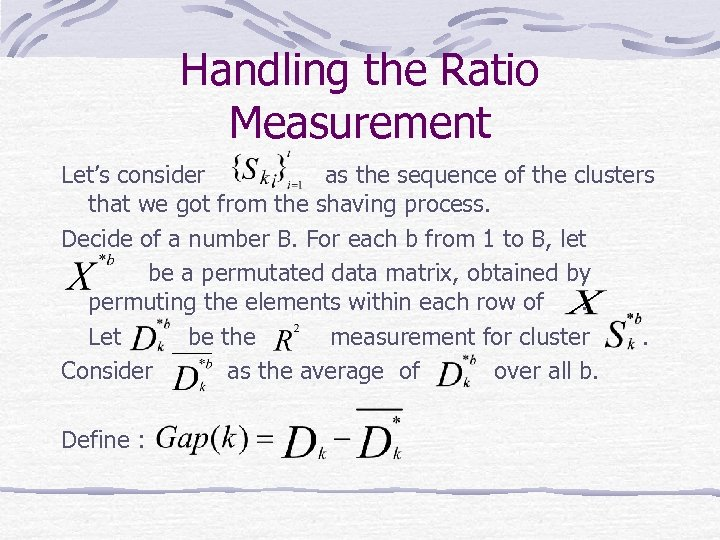Handling the Ratio Measurement Let’s consider as the sequence of the clusters that we got from the shaving process. Decide of a number B. For each b from 1 to B, let be a permutated data matrix, obtained by permuting the elements within each row of. Let be the measurement for cluster. Consider as the average of over all b. Define :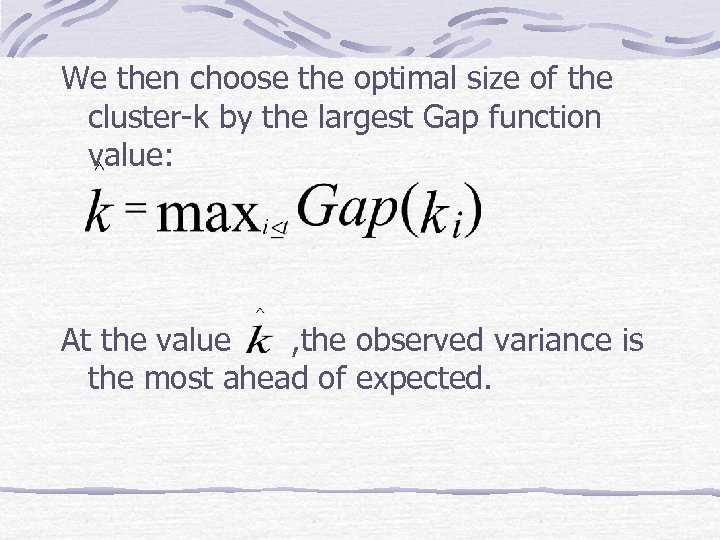We then choose the optimal size of the cluster-k by the largest Gap function value: At the value , the observed variance is the most ahead of expected.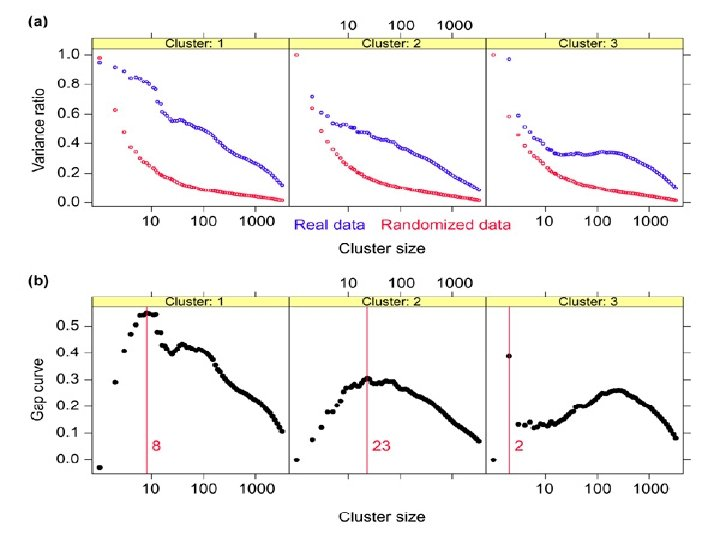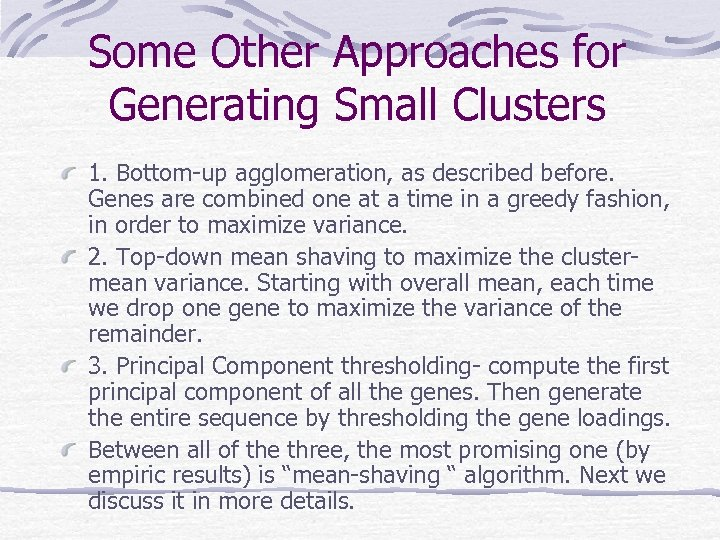Some Other Approaches for Generating Small Clusters 1. Bottom-up agglomeration, as described before. Genes are combined one at a time in a greedy fashion, in order to maximize variance. 2. Top-down mean shaving to maximize the clustermean variance. Starting with overall mean, each time we drop one gene to maximize the variance of the remainder. 3. Principal Component thresholding- compute the first principal component of all the genes. Then generate the entire sequence by thresholding the gene loadings. Between all of the three, the most promising one (by empiric results) is “mean-shaving “ algorithm. Next we discuss it in more details.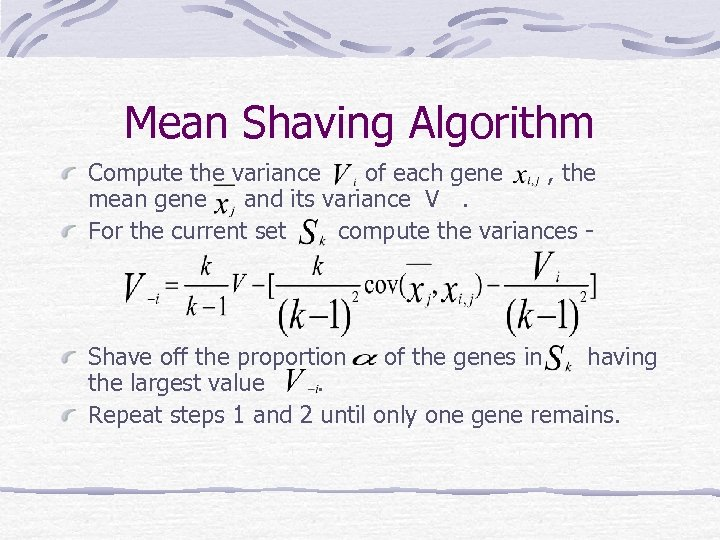Mean Shaving Algorithm Compute the variance of each gene , the mean gene and its variance V. For the current set compute the variances - Shave off the proportion of the genes in having the largest value. Repeat steps 1 and 2 until only one gene remains.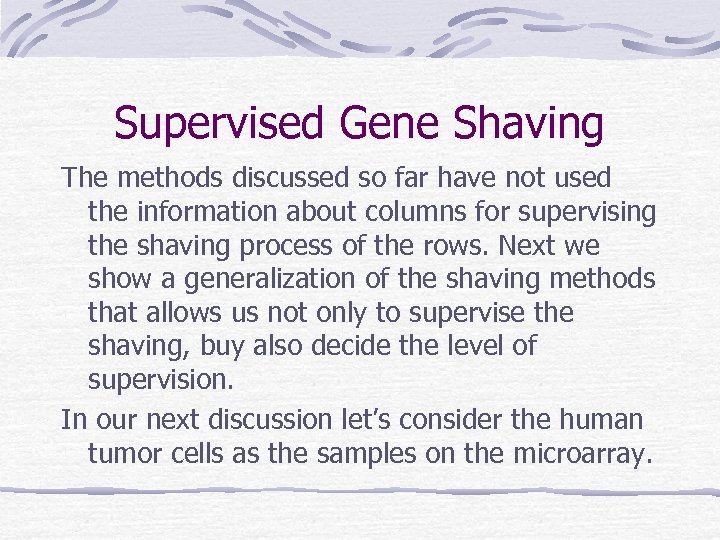Supervised Gene Shaving The methods discussed so far have not used the information about columns for supervising the shaving process of the rows. Next we show a generalization of the shaving methods that allows us not only to supervise the shaving, buy also decide the level of supervision. In our next discussion let’s consider the human tumor cells as the samples on the microarray.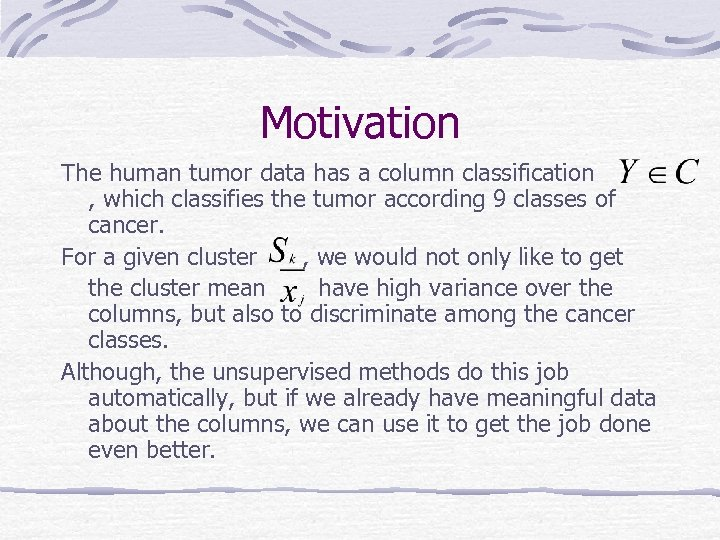Motivation The human tumor data has a column classification , which classifies the tumor according 9 classes of cancer. For a given cluster , we would not only like to get the cluster mean have high variance over the columns, but also to discriminate among the cancer classes. Although, the unsupervised methods do this job automatically, but if we already have meaningful data about the columns, we can use it to get the job done even better.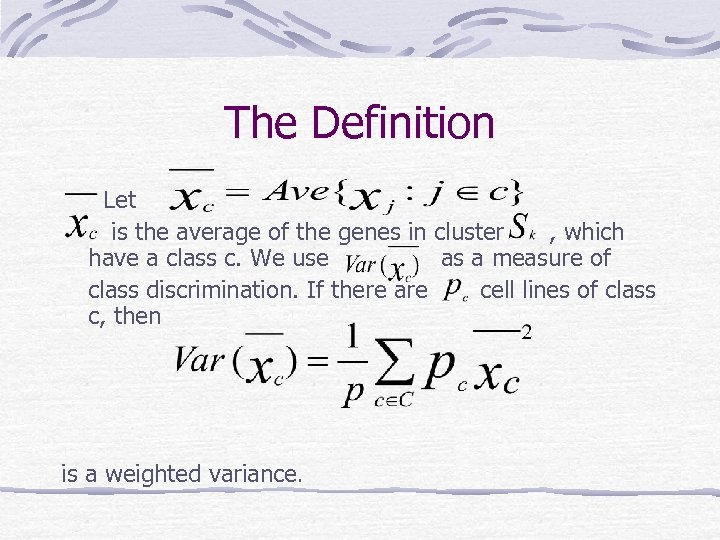The Definition Let is the average of the genes in cluster , which have a class c. We use as a measure of class discrimination. If there are cell lines of class c, then is a weighted variance.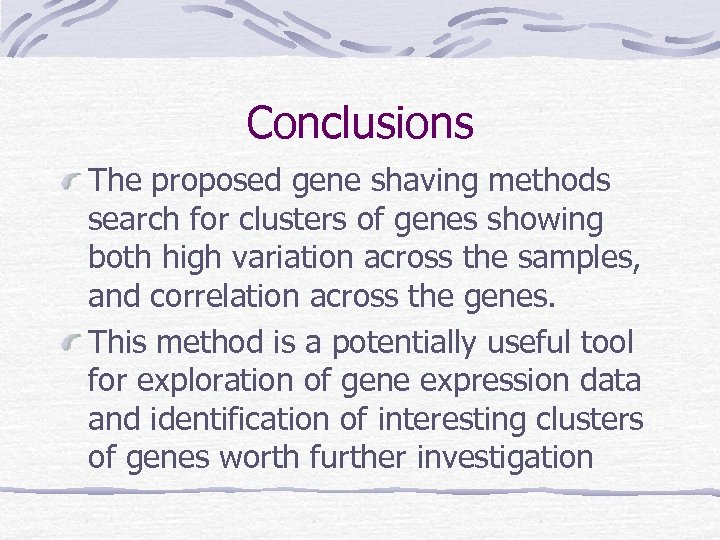Conclusions The proposed gene shaving methods search for clusters of genes showing both high variation across the samples, and correlation across the genes. This method is a potentially useful tool for exploration of gene expression data and identification of interesting clusters of genes worth further investigationRegularization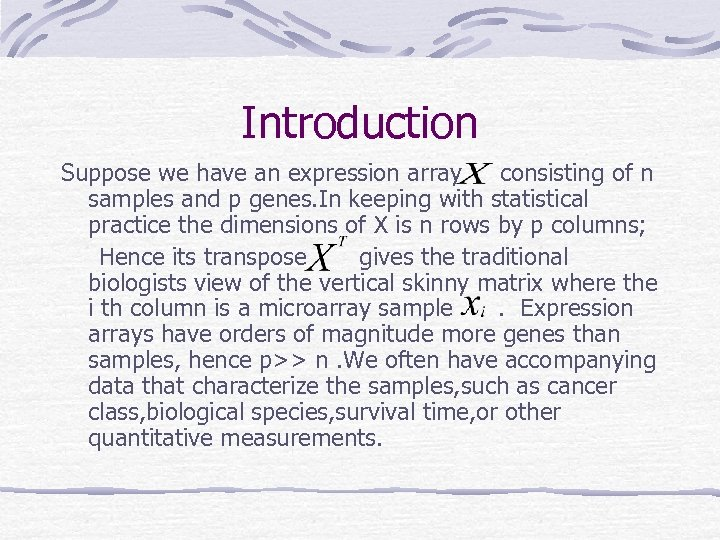Introduction Suppose we have an expression array consisting of n samples and p genes. In keeping with statistical practice the dimensions of X is n rows by p columns; Hence its transpose gives the traditional biologists view of the vertical skinny matrix where the i th column is a microarray sample. Expression arrays have orders of magnitude more genes than samples, hence p>> n. We often have accompanying data that characterize the samples, such as cancer class, biological species, survival time, or other quantitative measurements.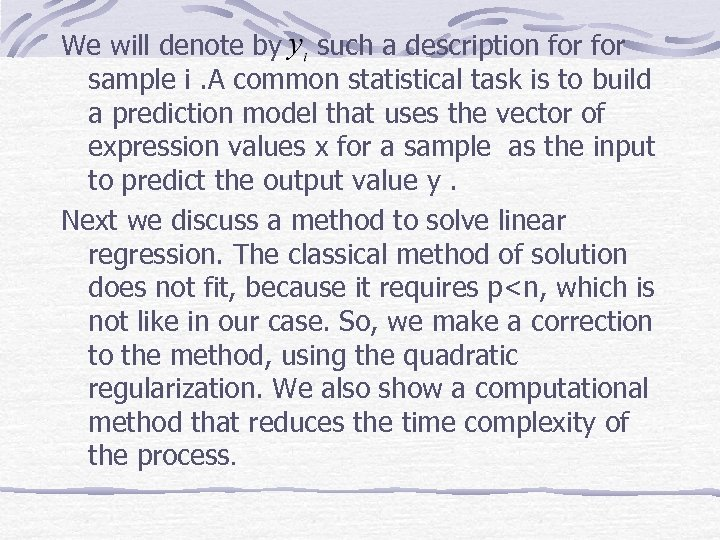We will denote by such a description for sample i. A common statistical task is to build a prediction model that uses the vector of expression values x for a sample as the input to predict the output value y. Next we discuss a method to solve linear regression. The classical method of solution does not fit, because it requires p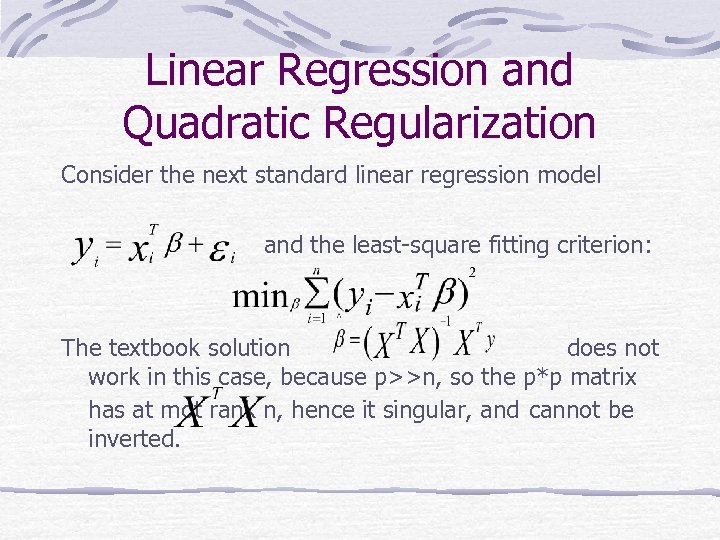Linear Regression and Quadratic Regularization Consider the next standard linear regression model and the least-square fitting criterion: The textbook solution does not work in this case, because p>>n, so the p*p matrix has at mot rank n, hence it singular, and cannot be inverted.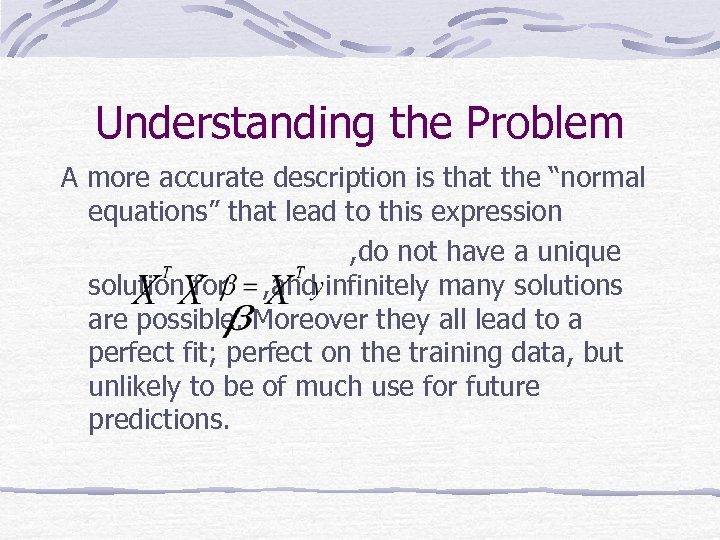Understanding the Problem A more accurate description is that the “normal equations” that lead to this expression , do not have a unique solution for , and infinitely many solutions are possible. Moreover they all lead to a perfect fit; perfect on the training data, but unlikely to be of much use for future predictions.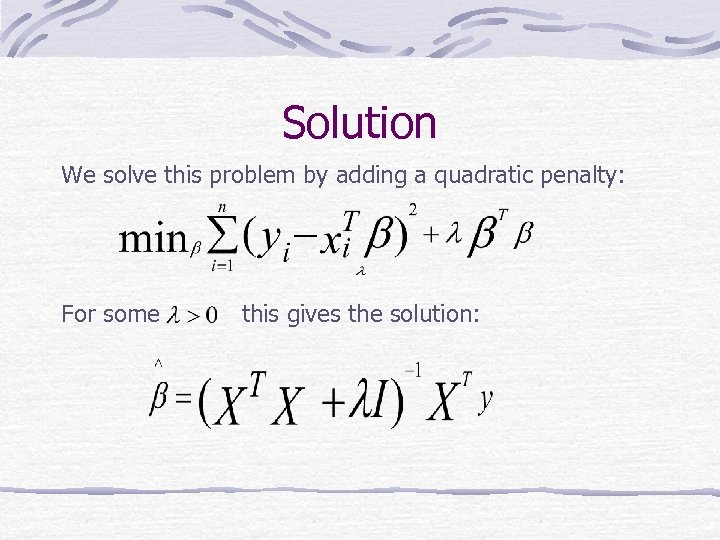Solution We solve this problem by adding a quadratic penalty: For some this gives the solution: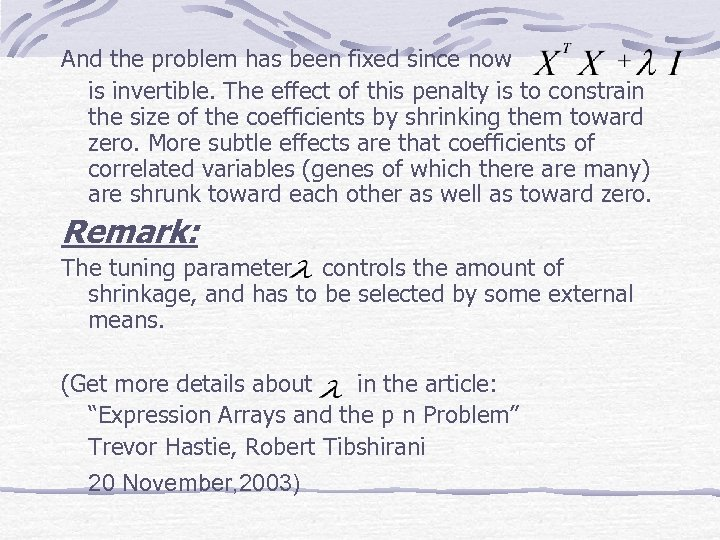And the problem has been fixed since now is invertible. The effect of this penalty is to constrain the size of the coefficients by shrinking them toward zero. More subtle effects are that coefficients of correlated variables (genes of which there are many) are shrunk toward each other as well as toward zero. Remark: The tuning parameter controls the amount of shrinkage, and has to be selected by some external means. (Get more details about in the article: “Expression Arrays and the p n Problem” Trevor Hastie, Robert Tibshirani 20 November, 2003)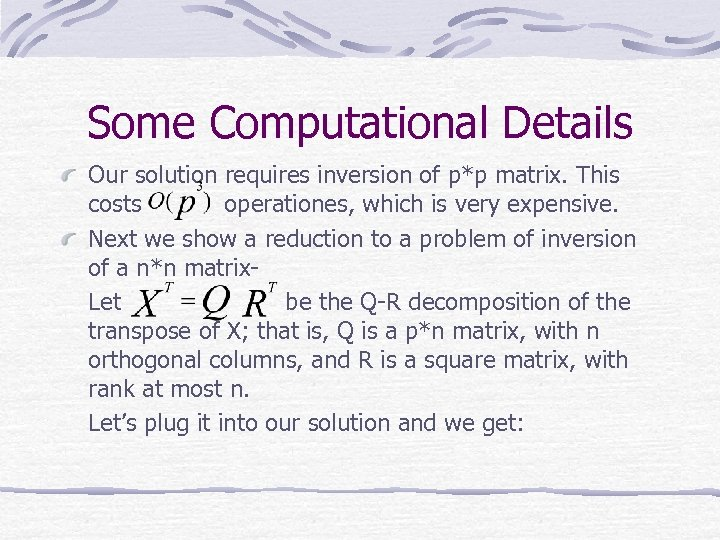Some Computational Details Our solution requires inversion of p*p matrix. This costs operationes, which is very expensive. Next we show a reduction to a problem of inversion of a n*n matrix. Let be the Q-R decomposition of the transpose of X; that is, Q is a p*n matrix, with n orthogonal columns, and R is a square matrix, with rank at most n. Let’s plug it into our solution and we get: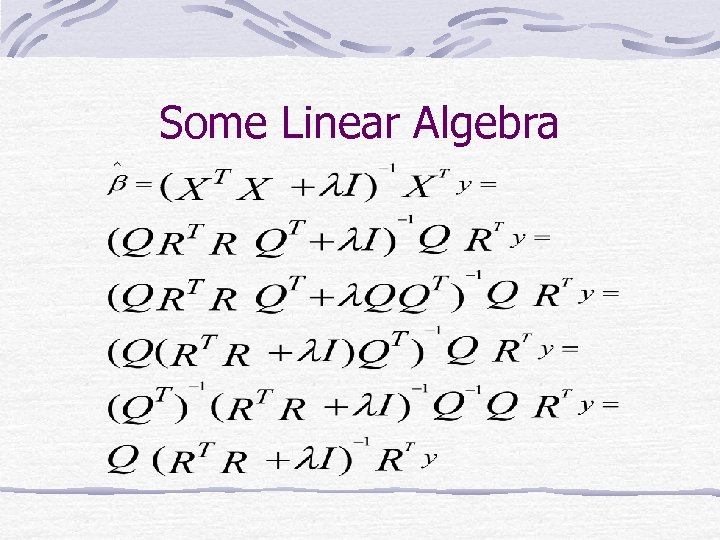Some Linear Algebra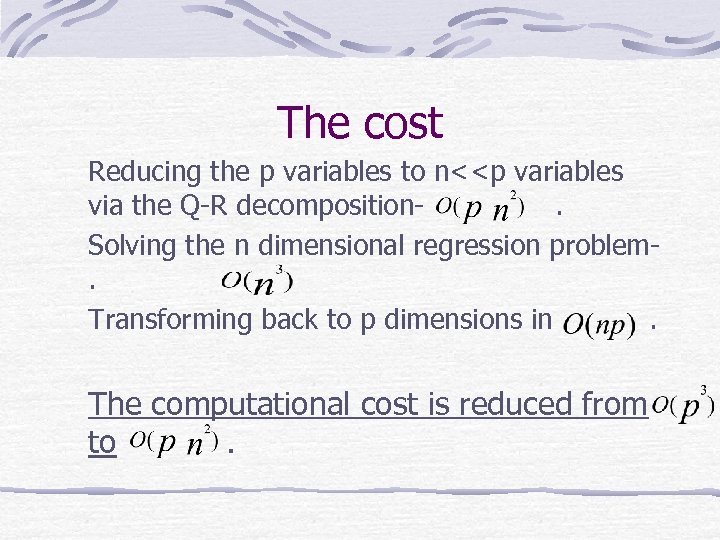The cost Reducing the p variables to n<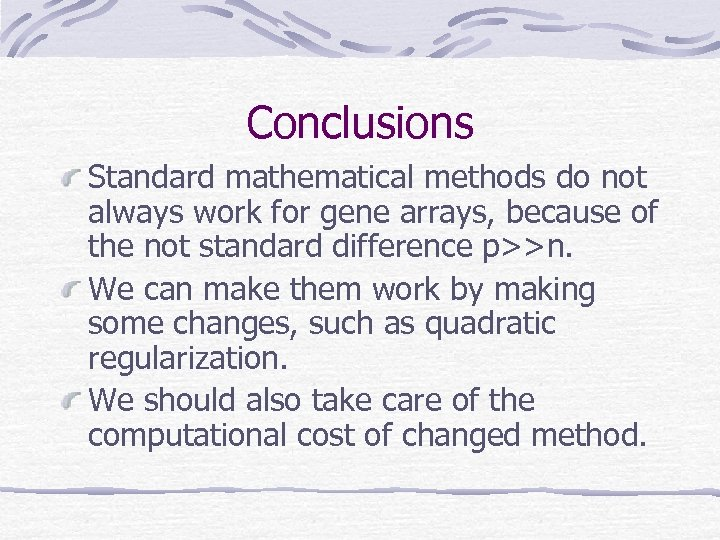Conclusions Standard mathematical methods do not always work for gene arrays, because of the not standard difference p>>n. We can make them work by making some changes, such as quadratic regularization. We should also take care of the computational cost of changed method.END